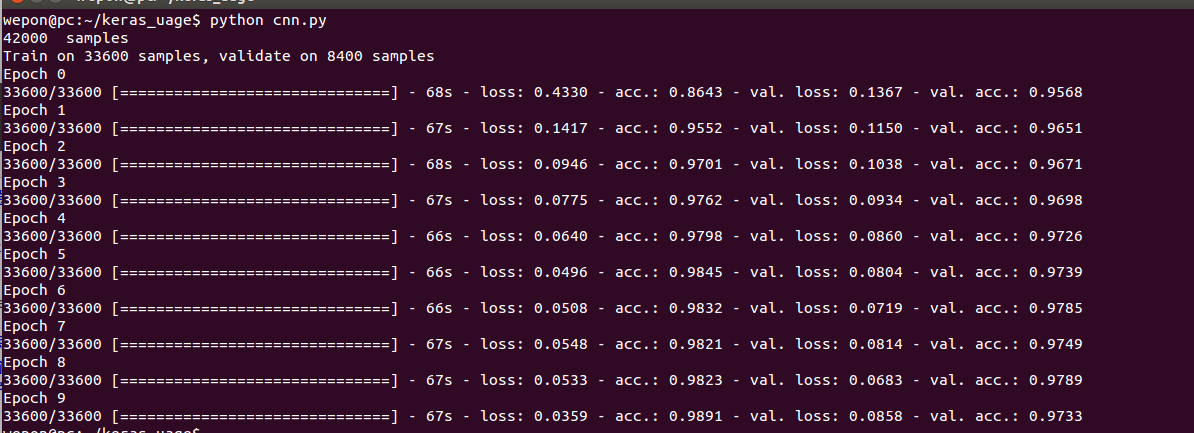# DeepLearning tutorial（6）易用的深度学习框架Keras简介

## 1. Keras简介

Keras是基于Theano的一个深度学习框架，它的设计参考了Torch，用Python语言编写，是一个高度模块化的神经网络库，支持GPU和CPU。使用文档在这：http://keras.io/，这个框架貌似是刚刚火起来的，使用上的问题可以到github提issue:https://github.com/fchollet/keras

## 2. Keras里的模块介绍

• Optimizers

keras.optimizers.SGD(lr=0.01, momentum=0.9, decay=0.9, nesterov=False)

上面的代码是SGD的使用方法，lr表示学习速率,momentum表示动量项，decay是学习速率的衰减系数(每个epoch衰减一次),Nesterov的值是False或者True，表示使不使用Nesterov momentum。其他的请参考文档。

• Objectives

这是目标函数模块，keras提供了mean_squared_error，mean_absolute_error
，squared_hinge，hinge，binary_crossentropy，categorical_crossentropy这几种目标函数。

这里binary_crossentropy 和 categorical_crossentropy也就是常说的logloss.

• Activations

• Initializations

这是参数初始化模块，在添加layer的时候调用init进行初始化。keras提供了uniform、lecun_uniform、normal、orthogonal、zero、glorot_normal、he_normal这几种。

• layers

其中core里面包含了flatten(CNN的全连接层之前需要把二维特征图flatten成为一维的)、reshape（CNN输入时将一维的向量弄成二维的）、dense(就是隐藏层，dense是稠密的意思),还有其他的就不介绍了。convolutional层基本就是Theano的Convolution2D的封装。

• Preprocessing

这是预处理模块，包括序列数据的处理，文本数据的处理，图像数据的处理。重点看一下图像数据的处理，keras提供了ImageDataGenerator函数,实现data augmentation，数据集扩增，对图像做一些弹性变换，比如水平翻转，垂直翻转，旋转等。

• Models

这是最主要的模块，模型。上面定义了各种基本组件，model是将它们组合起来，下面通过一个实例来说明。

## 3.一个实例：用CNN分类Mnist

• 数据下载

Mnist数据在其官网上有提供，但是不是图像格式的，因为我们通常都是直接处理图像，为了以后程序能复用，我把它弄成图像格式的，这里可以下载：http://pan.baidu.com/s/1qCdS6，共有42000张图片。

• 读取图片数据

keras要求输入的数据格式是numpy.array类型（numpy是一个python的数值计算的库），所以需要写一个脚本来读入mnist图像，保存为一个四维的data，还有一个一维的label，代码：

#coding:utf-8
"""
Author:wepon
Source:https://github.com/wepe
file:data.py
"""

import os
from PIL import Image
import numpy as np

#读取文件夹mnist下的42000张图片，图片为灰度图，所以为1通道，
#如果是将彩色图作为输入,则将1替换为3，并且data[i,:,:,:] = arr改为data[i,:,:,:] = [arr[:,:,0],arr[:,:,1],arr[:,:,2]]
data = np.empty((42000,1,28,28),dtype="float32")
label = np.empty((42000,),dtype="uint8")

imgs = os.listdir("./mnist")
num = len(imgs)
for i in range(num):
img = Image.open("./mnist/"+imgs[i])
arr = np.asarray(img,dtype="float32")
data[i,:,:,:] = arr
label[i] = int(imgs[i].split('.'))
return data,label
• 构建CNN，训练

短短二十多行代码，构建一个三个卷积层的CNN，直接读下面的代码吧，有注释，很容易读懂：

#导入各种用到的模块组件
from __future__ import absolute_import
from __future__ import print_function
from keras.preprocessing.image import ImageDataGenerator
from keras.models import Sequential
from keras.layers.core import Dense, Dropout, Activation, Flatten
from keras.layers.advanced_activations import PReLU
from keras.layers.convolutional import Convolution2D, MaxPooling2D
from keras.utils import np_utils, generic_utils
from six.moves import range
from data import load_data

#加载数据
data, label = load_data()
print(data.shape, ' samples')

#label为0~9共10个类别，keras要求格式为binary class matrices,转化一下，直接调用keras提供的这个函数
label = np_utils.to_categorical(label, 10)

###############
#开始建立CNN模型
###############

#生成一个model
model = Sequential()

#第一个卷积层，4个卷积核，每个卷积核大小5*5。1表示输入的图片的通道,灰度图为1通道。
#border_mode可以是valid或者full，具体看这里说明：http://deeplearning.net/software/theano/library/tensor/nnet/conv.html#theano.tensor.nnet.conv.conv2d
#激活函数用tanh
model.add(Convolution2D(4, 1, 5, 5, border_mode='valid'))

#第二个卷积层，8个卷积核，每个卷积核大小3*3。4表示输入的特征图个数，等于上一层的卷积核个数
#激活函数用tanh
#采用maxpooling，poolsize为(2,2)
model.add(Convolution2D(8,4, 3, 3, border_mode='valid'))

#第三个卷积层，16个卷积核，每个卷积核大小3*3
#激活函数用tanh
#采用maxpooling，poolsize为(2,2)
model.add(Convolution2D(16, 8, 3, 3, border_mode='valid'))

#全连接层，先将前一层输出的二维特征图flatten为一维的。
#Dense就是隐藏层。16就是上一层输出的特征图个数。4是根据每个卷积层计算出来的：(28-5+1)得到24,(24-3+1)/2得到11，(11-3+1)/2得到4
#全连接有128个神经元节点,初始化方式为normal

#Softmax分类，输出是10类别

#############
#开始训练模型
##############
#使用SGD + momentum
#model.compile里的参数loss就是损失函数(目标函数)
sgd = SGD(l2=0.0,lr=0.05, decay=1e-6, momentum=0.9, nesterov=True)
model.compile(loss='categorical_crossentropy', optimizer=sgd,class_mode="categorical")

#调用fit方法，就是一个训练过程. 训练的epoch数设为10，batch_size为100．
#数据经过随机打乱shuffle=True。verbose=1，训练过程中输出的信息，0、1、2三种方式都可以，无关紧要。show_accuracy=True，训练时每一个epoch都输出accuracy。
#validation_split=0.2，将20%的数据作为验证集。
model.fit(data, label, batch_size=100,nb_epoch=10,shuffle=True,verbose=1,show_accuracy=True,validation_split=0.2)
• 代码使用与结果